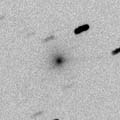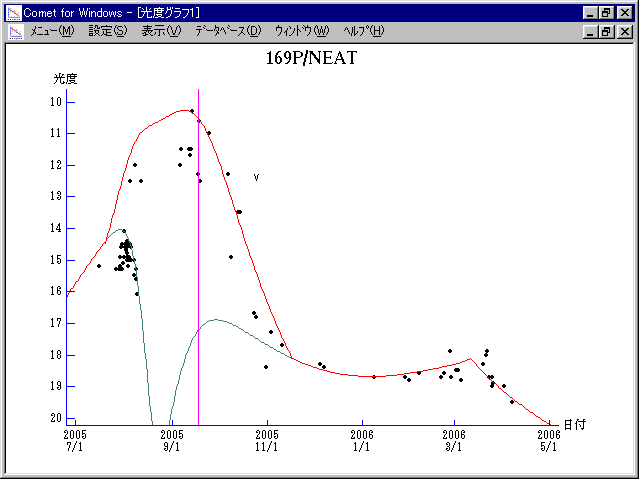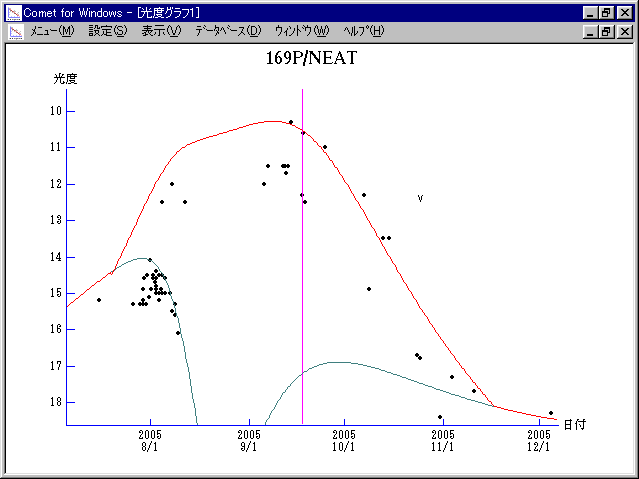# 169P/NEAT (2005)###Pictures###Orbital Elements

```                    Epoch = 2005 Aug. 18.0 TT
T = 2005 Sept.17.8587 TT         Peri. = 217.9272
e = 0.767520                     Node  = 176.2462  2000.0
q = 0.605325 AU                  Incl. =  11.3189
a =  2.603775 AU    n = 0.2345843    P =   4.202 years
```

###Finding Charts###Magnitudes Graph

```        H = 15.3  G = 0.15                [   ,-60]  (             - 2005 July 19)
m1 = 15.8 + 5 log d + 20.0 log r  [-60, 60]  (2005 July 19 - 2005 Nov. 16)
H = 15.3  G = 0.15                [ 60,   ]  (2005 Nov. 16 -             )

* Green curve is:  H = 15.3  G = 0.15
```##### The orbital elements are published on IAUC 8578. The charts are made with StellaNavigator Ver.2.0 for Windows (AstroArts / ASCII). The magnitudes graphs are made with Comet for Windows.